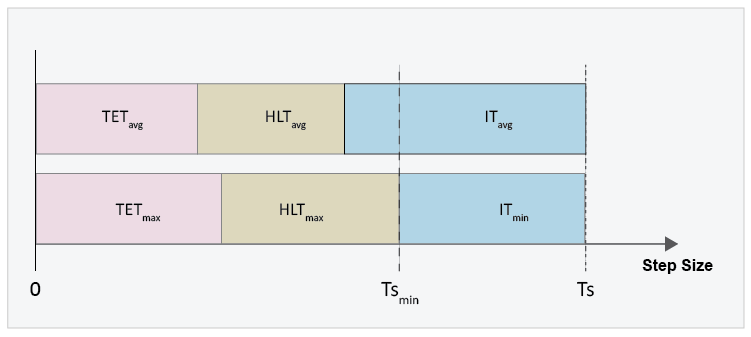## Estimate Computation Costs

Estimating computational cost helps you to determine if your model is likely to cause an overrun when you simulate it on your real-time processor. Computational cost is the execution time per time step during simulation. To estimate the time that it takes for your model to execute on real-time hardware, estimate the simulation execution-time budget for your real-time target machine.

To estimate the simulation execution-time, first, measure the execution time of desktop simulation for a particular model. Then determine the average execution time per time step on the real-time target machine for the same model. Knowing how these execution times compare for one model means that you can estimate execution time on the real-time target machine from desktop simulation execution time when you test other models. Having an estimate for the execution-time budget helps you to choose a feasible combination of solver settings for fixed-step, fixed-cost simulation.

During each time step, the real-time target machine must perform the procedures that the figure shows.The equation for determining the minimum step size to specify for the fixed-step solver to avoid simulation overrun is

`$T{s}_{\mathrm{min}}=TE{T}_{\mathrm{max}}+HL{T}_{\mathrm{max}},$`

where

• TET is the task execution time. Task execution time involves calculating the simulation results for the time step, processing inputs from and writing outputs to the development computer, and performing general computing tasks such as buffering data and accessing memory.

• HLT is the hardware latency time. Hardware latency time includes scheduling, interrupt, and input/output (I/O) latency.

• Tsmin is the minimum step size.

If the time that it takes for the target machine to execute the simulation and handle latency processes is less than the specified time step, the processor remains idle during the remainder of the step. That is,

`$Ts=TE{T}_{\mathrm{max}}+HL{T}_{\mathrm{max}}+IT,$`

where

• Ts is the step size that you specify for the fixed-step solver.

• IT is the idle time.

This equation can be rearranged as:

`$TE{T}_{\mathrm{max}}=Ts-HL{T}_{\mathrm{max}}-IT,$`

The task execution, hardware latency, and idle times vary, but you can implement a safety margin by specifying the idle time in the budget calculation as a function of the step size for the fixed-step solver. For example, if you specify a step size of 1e-5 for the solver, and you want a 20% safety margin, then IT = (0.2)*(1e-5).

Therefore, the amount of time available for simulation execution can be calculated as follows:

`$TE{T}_{\mathrm{max}}=Ts-HL{T}_{\mathrm{max}}-\left[\left(SMT\right)*\left(Ts\right)\right],$`

where

• SMT is the desired safety margin, specified as a percent.